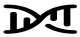# Generalized Method of Moments (GMM) estimator

Generalized Method of Moments (GMM) is an estimation technique that is helpful where the distribution of the data maybe unknown and therefore alternative estimation techniques such as maximum likelihood are not applicable. For a given model, we specify a certain number of moment conditions, which are functions of the model parameters and the data, such that their expectation is zero at the parameters' true values. The GMM estimator of a linear IV model has been applied to individual-level data in Economics since the 1980s.

In the context of IV estimation, we usually specify 1 moment condition per IV. The GMM method then minimizes a certain norm of the sample averages of the moment conditions. For identification we require at least as many IVs as there are parameters to be estimated. For binary outcomes, GMM estimation of structural mean models can be used to estimate parameters such as the causal risk ratio in MR studies using individual-level data.